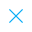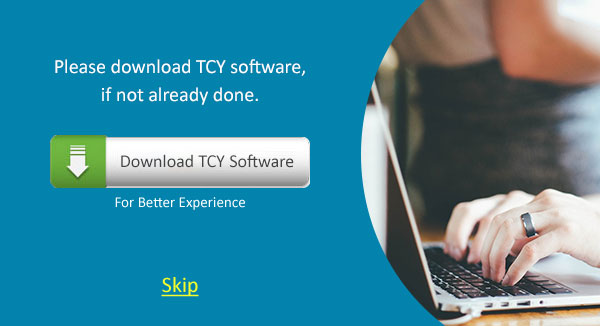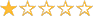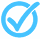Call Support +91-85588-96644

Keep me logged in
You can't leave Captcha Code empty
By submitting this form, you agree to the Terms & Privacy Policy.
ORTests given

ORLinear Equations| By TCY

Topics Coverd:GraphsCross MultiplicationMultiplicationMathEquationsTrigonometryLinear EquationsSurface AreaSolidsCirclesCubeMathMath Sample PapersMath Test Papers

Description:
Get Downloadable Notes for Graphs, Cross Multiplication, Multiplication, Math, Equations, Trigonometry, Linear Equations, Surface Area, Solids, Circles, Cube, Math, Math Sample Papers, Math Test Papers
Keywords: